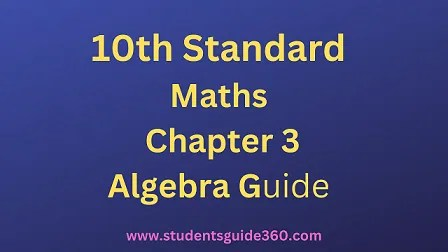# 10th Maths Guide Chapter 3 Algebra Exercise 3.7

10th Standard Maths Chapter 3 Algebra Samacheer Kalvi Guide Exercise 3.7 Book Back Answers Solutions. TN 10th SSLC Samacheer Kalvi Guide. 10th All Subject Guide – Click Here. Class 1 to 12 All Subject Book Back Answers – Click Here## 10th Maths Guide Chapter 3 Algebra Exercise 3.7

### 1. Find the square root of the following rational expressions.Solution:### 2. Find the square root of the following

• (i) 4×2 + 20x + 25
• (ii) 9×2 – 24xy + 30xz – 40yz + 25z2 + 16y2
• (iii) 1 + 1×6+2×3
• (iv) (4×2 – 9x + 2) (7×2 – 13x – 2) (28×2 – 3x – 1)
• (v) ( 2×2 + 17/6x + 1) (3/2×^2 + 4x + 2)(4/3×2+11/3x + 2)

Solution: